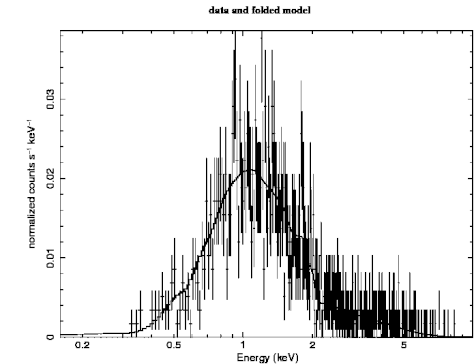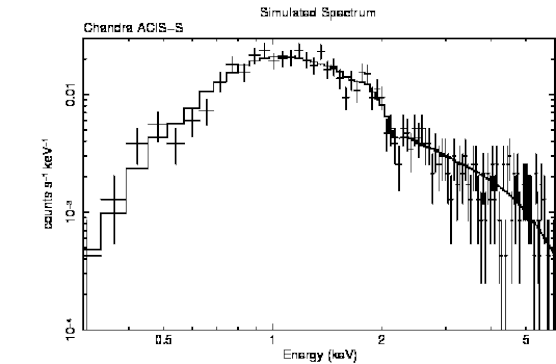Next: Markov Chain Monte Carlo Up: Walks through XSPEC Previous: Using XSPEC to Simulate

# Producing Plots: Modifying the Defaults

The final results of using XSPEC are usually one or more tables containing confidence ranges and fit statistics, and one or more plots showing the fits themselves. So far, the plots shown have generally used the default settings, but it is possible to edit plots to improve their appearance.

The plotting package used by XSPEC is PGPLOT, which is comprised of a library of low-level tasks. At a higher level is QDP/PLT, the interactive program that forms the interface between the XSPEC user and PGPLOT. QDP/PLT has its own manual; it also comes with on-line help. Here, we show how to make some of the most common modifications to plots.

In this example, we'll take the simulated Chandra spectrum and make a better plot. Figure 4.9 shows the basic data and folded model plot. The only additional changes we have made to this plot are to increase the line widths to make them print better. We made this plot as follows:

```XSPEC12>setplot energy
XSPEC12>iplot data
PLT>lwidth 3
PLT>lwidth 3 on 1..2
PLT>time off
PLT>hard figi.png/ps
```

The first lwidth command increases the line widths on the frame while the second increases it on the data and model. The time off command just removes a username and time stamp from the bottom right of the plot. The hard command makes a ``hardcopy'', in this case a PostScript file. Before looking at other PLT commands we can use to modify the plot there is one more thing we can try at the XSPEC level. The current bin sizes are much smaller than the resolution so we may as well combine bins in the plot (but not in the fitting) to make it clearer.

```XSPEC12>setplot rebin 100 4
```

Combines four spectral bins into one. The setplot rebin command combines bins till either a S/N of the first argument is reached or the number of bins in the second argument have been combined. We do an iplot again then do the following modifications:

```PLT> viewport 0.2 0.2 0.8 0.7
```

The first thing we'll do is change the aspect ratio of the box that contains the plot (viewport in QDP terminology). The viewport is defined as the coordinates of the lower left and upper right corners of the page. The units are such that the full width and height of the page are unity. The labels fall outside the viewport, so if the full viewport were specified, only the plot would appear. The default box has a viewport with corners at (0.1, 0.1) and (0.9, 0.9). For our purposes, we want a viewport with corners at (0.2, 0.2) and (0.8, 0.7): with this size and shape, the hardcopy will fit nicely on the page and not have to be reduced for photocopying.Next we want to change some of the labels:

```PLT> label top Simulated Spectrum
PLT> label file Chandra ACIS-S
PLT> label y counts s\u-1\d keV\u-1\d
```

To get help on a PLT command, just type help followed by the name of the command. Note that PLT commands can be abbreviated, just like XSPEC commands. To see the results of changing the viewport and the labels, just enter the command plot.

The two changes we want to make next are to rescale the axes and to change the y-axis to a logarithmic scale. The commands for these changes also are straightforward: the rescale command takes the minimum and maximum values as its arguments, while the log command takes x or y as arguments:

```PLT> rescale x 0.3 6.0
PLT> rescale y 1.0e-4 0.03
PLT> log y
PLT> plot
```

To revert to a linear scale, use the command log off y. The only remaining extra change is to reduce the size of the characters. This is done using the csize command, which takes the normalization as its argument. One (1) will not change the size, a number less than one will reduce it and a number bigger than one will increase it.```PLT> csize 0.8
PLT> plot
```

We make the PostScript file and also save the plot information using the wenv command that, in this case, writes files figj.qdp and figj.pco containing the plot data and commands, respectively.

```PLT> hardcopy figj.png/ps
PLT> wenv figj
PLT> quit
```

The result of all this manipulation is shown proudly in Figure 4.10.Next: Markov Chain Monte Carlo Up: Walks through XSPEC Previous: Using XSPEC to Simulate